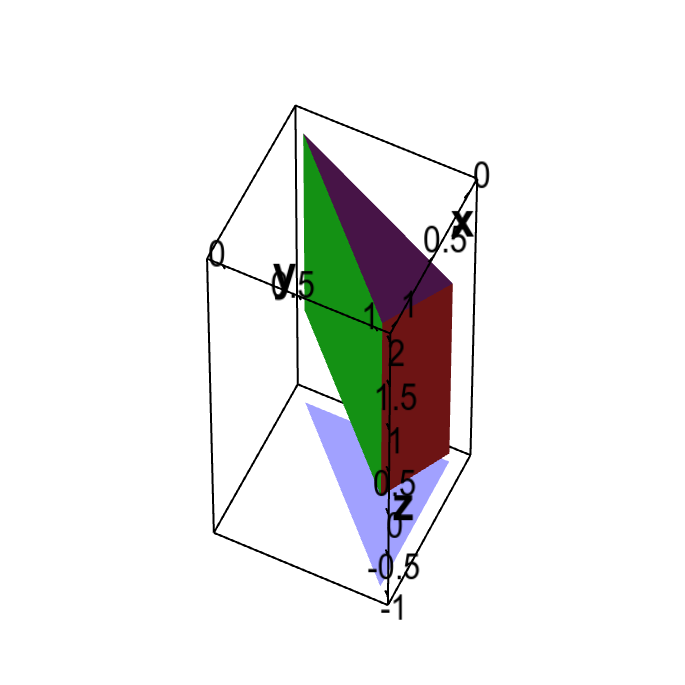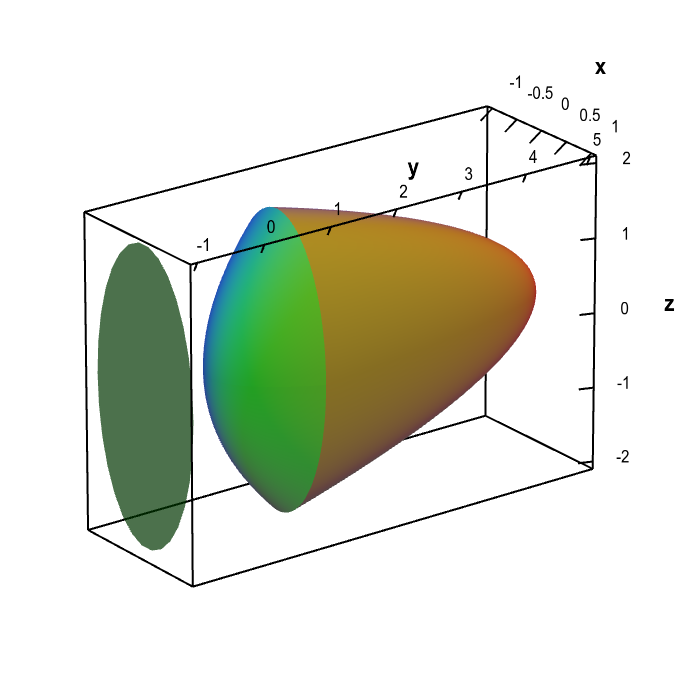# Math Insight

### The shadow method for determining triple integral bounds

Although we define triple integrals using a Riemann sum, we usually evaluate triple integrals by turning them into iterated integrals involving three single integrals. One tricky part of triple integrals is describing the three-dimensional regions of integration and the resulting bounds on the iterated integrals. Forming double iterated integrals is easier because one can draw the domain and label all the edges and corners, which makes determining the bounds more tractable. Three-dimensional regions are much more difficult to visualize or draw, which can make the prospect of determining integration bounds a formidable task.

Fortunately, there are ways to reduce a triple integral into a double integral combined with a single integral. One such method is one we call the “shadow method,” which we describe here. A related method is one we call the “cross section method” which we describe on another page.

In the shadow method, you imagine there is a light source, such as the sun, positioned far away along one of the coordinate axis (such as the positive $z$-axis). We'll think of this sun as being straight up in the sky and think of the chosen coordinate axis as though it were vertical.

As this sun is shining on the three-dimesional region $\dlv$ of our integral, it is casting a shadow onto the flat ground below the region, i.e., on a plane perpendicular to the axis the sun is coming from. This shadow is a two-dimensional region, and we turn the triple integral over $\dlv$ into a double integral over the shadow. Inside the double integral, we still need to include a single integral in the third “vertical” variable, where this variable ranges from the bottom of $\dlv$ to its top. If, for example, we had chosen $z$ to be the “vertical” variable where the sun is coming from, the shadow method for integrating a function $f$ over $\dlv$ would be of the form \begin{gather*} \iiint_{\dlv}f(x,y,z)dV = \iint_{\text{shadow}} \left( \int_{\text{bottom}(x,y)}^{\text{top}(x,y)} f(x,y,z) dz\right) dx\,dy. \end{gather*} But, we don't need to have the sun coming from the $z$ direction. It might be easier to let the sun be in the $x$ direction, giving an integral of the form \begin{gather*} \iiint_{\dlv}f(x,y,z) dV= \iint_{\text{shadow}} \left( \int_{\text{bottom}(y,z)}^{\text{top}(y,z)} f(x,y,z) dx\right) dy\,dz, \end{gather*} or let the sun being in the $y$ direction, giving an integral of the form \begin{gather*} \iiint_{\dlv}f(x,y,z)dV = \iint_{\text{shadow}} \left( \int_{\text{bottom}(x,z)}^{\text{top}(x,z)} f(x,y,z) dy\right) dx\,dz. \end{gather*} We can choose the axis we use for the “vertical” direction based on the shape of the region $\dlv$.

The use of the shadow method is best illustrated by examples.

#### Example 1

Let $\dlv$ be the three-dimesional region bounded by the vertical planes $x=0$, $y=1$, and $x=y$ as well as the angled planes $z=2+x-y$ and $z=x-y$. Let $f(x,y,z)=xy$ and compute the integral of $f$ over $\dlv$.

Solution: Given that there are three planes parallel to the $z$-axis, we'll use the $z$ direction as the vertical direction. We'll think of the sun as coming from that direction and casting a shadow below it. As shown by the below figure, those three vertical planes cause $\dlv$ to cast a triangular shadow.Region bounded by planes demonstrating the shadow method. The region $\dlv$ is bounded by the vertical planes $x=0$, $y=1$, and $x=y$ and the angled planes $z=2+x-y$ and $z=x-y$. If the sun is viewed to be in the direction of the positive $z$-axis, the resulting shadow on a plane perpendicular to the $z$-axis is a triangle, as illustrated by the blue triangle below the region.

According to the shadow method, we need to integrate $x$ and $y$ over this shadow. Then, for each point $(x,y)$ in the shadow, we need to integrate $z$ from the bottom to the top of $\dlv$. The bottom and top are formed by the angled planes, so the range of $z$ for each point in the shadow is \begin{gather*} x-y \lt z \lt 2+x-y. \end{gather*} The inner integral is therefore \begin{gather*} \int_{\text{bottom}(x,y)}^{\text{top}(x,y)} f(x,y,z) dz = \int_{x-y}^{2+x-y}f(x,y,z)dz. \end{gather*}

The outer double integral is an integral over the shadow. The shadow is the maximal extend of $\dlv$ in the $x$ and $y$ directions. For this example, the shadow is fairly simple, since the extent of $\dlv$ is determined by the three vertical planes $x=0$, $y=1$ and $x=y$. In any plane parallel to the $xy$-plane, these planes become lines given by the same equations (as the original equations don't contain a $z$). The shadow is the triangle bounded by the lines $x=0$, $y=1$ and $x=y$.

To find bounds for the double integral over the shadow, we'll let $x$ be the inner integral. For any value of $y$, $x$ ranges from $x=0$ to $x=y$ so the range of $x$ is \begin{gather*} 0 \lt x \lt y. \end{gather*} The maximum range of $y$ is shadow is \begin{gather*} 0 \lt y \lt 1. \end{gather*} Therefore, the double integral over the shadow is of the form \begin{gather*} \iint_{\text{shadow}} \cdots dx\,dy = \int_0^1 \int_0^y \cdots dx\,dy. \end{gather*}

Putting all the limits of integration together and inserting the integrand $f(x,y,z)=xy$, we calculate the integral: \begin{align*} \iiint_{\dlv}f(x,y,z)dV &=\int_0^1 \int_0^y \int_{x-y}^{2+x-y} xy\, dz\,dx\,dy\\ &= \int_0^1 \int_0^y xyz \bigg|_{z=x-y}^{z=2+x-y} dx\,dy\\ &= \int_0^1 \int_0^y 2xy dx\,dy\\ &= \int_0^1 x^2y \bigg|_{x=0}^{x=y}dy\\ &= \int_0^1 y^3 dy = \frac{y^4}{4}\bigg|_0^1 = \frac{1}{4}. \end{align*}

#### Example 2

Let $\rho(x,y,z)$ be the charge density at the point $(x,y,z)$ inside an object $\dlv$ bounded by the elliptic paraboloids $y=5-4 x^2-z^2$ and $y=x^2+z^2/4$. Set up the integral giving the total charge inside $\dlv$.

Solution: The total charge is the integral of the charge density $\rho$ over the region $\dlv$: \begin{gather*} \text{total charge} = \iiint_{\dlv} \rho(x,y,z) \, dV. \end{gather*} Our task is to calculate the limits of integration determined by $\dlv$.

For this region, the shadow method will work well if we choose the $y$-axis as the “vertical” direction, i.e., the direction where the sun comes from. If the positive $y$ direction is upward, then one boundary, $y=5-4 x^2-z^2$, is a paraboloid opening downward that intersects the $y$-axis at $y=5$. The other boundary, $y=x^2+z^2/4$ is a paraboloid that opens upward and intersects the $y$-axis at the origin. Therefore, the bounded region $\dlv$ between the surfaces will be the region below $y=5-4 x^2-z^2$ and above $y=x^2+z^2/4$, i.e., the region described by $$x^2+\frac{z^2}{4} \le y \le 5-4 x^2-z^2,$$ as illustrated below. The two paraboloid surfaces are the top and bottom limits for the shadow method, \begin{gather*} \int_{\text{bottom}(x,z)}^{\text{top}(x,z)} \rho(x,y,z) dy = \int_{x^2+z^2/4}^{5-4 x^2-z^2}\rho(x,y,z)dy. \end{gather*}Region bounded by paraboloids demonstrating the shadow method. The region $\dlv$ is bounded by the elliptic paraboloids $y=5-4 x^2-z^2$ and $y=x^2+z^2/4$. If the sun is viewed to be in the direction of the positive $y$-axis, the shadow of $\dlv$ in a plane perpendicular to the $y$-axis is the region inside the ellipse $x^2+z^2/4=1$.

The remaining task is to determine the shadow of $\dlv$, illustrated by the ellipse, above. The widest part of $\dlv$ is where the two paraboloids intersect, i.e., where $$x^2+\frac{z^2}{4} = 5-4 x^2-z^2,$$ which can be written more simply as $$x^2+\frac{z^2}{4} = 1.$$ This boundary of the shadow is an ellipse with a semi-major axis of 2 and a semi-minor axis of 1. The shadow itself is the region $$x^2+\frac{z^2}{4} \le 1,$$ which includes the interior of the ellipse, shown below.

Since inside the ellipse, $x^2 \le 1- z^2/4$, the range of $x$ for each value of $z$ is $$-\sqrt{1-z^2/4} \le x \le \sqrt{1-z^2/4}.$$ The maximum range of $z$ is $-2 \le z \le 2$, so the integration limits for the shadow are \begin{gather*} \iint_{\text{shadow}} \cdots dx\,dz = \int_{-2}^2 \int_{-\sqrt{1-z^2/4}}^{\sqrt{1-z^2/4}} \cdots dx\,dz. \end{gather*}

Putting the top and bottom limits together with the shadow, we conclude that the total charge inside $\dlv$ is the integral \begin{align*} \text{total charge} &= \iiint_{\dlv} \rho(x,y,z) \, dV\\ &=\int_{-2}^2 \int_{-\sqrt{1-z^2/4}}^{\sqrt{1-z^2/4}}\int_{x^2+z^2/4}^{5-4 x^2-z^2}\rho(x,y,z)dy\,dx\,dz. \end{align*}